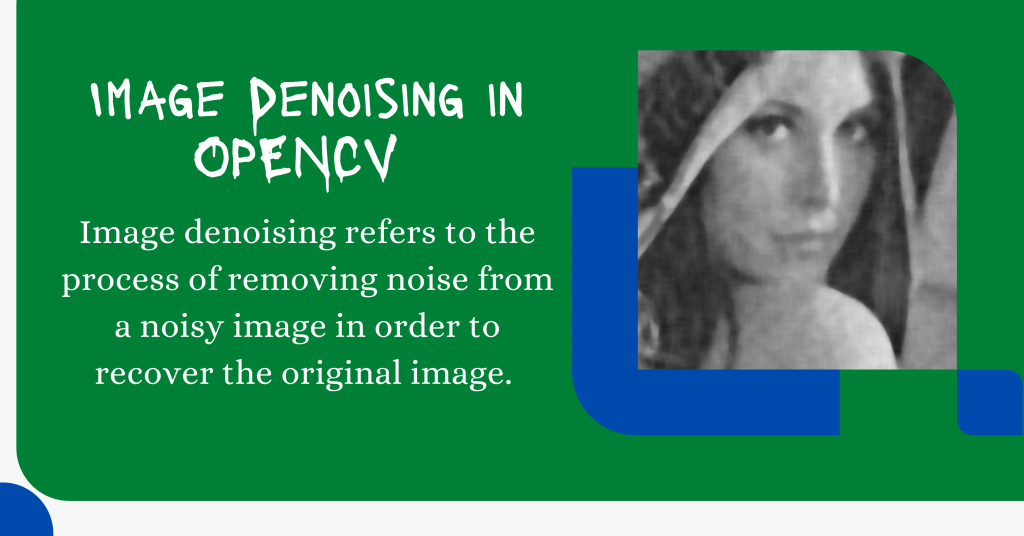# Denoising Images in Python – A Step-By-Step GuideIn this tutorial, we have used a machine-learning algorithm to denoise a noisy image by making use of Python as the programming language.

Let’s get straight to what image denoising is and how to implement the same in the coming sections.

Also read: Visualizing Colors In Images Using Histograms – Python OpenCV

## Introduction to Image Denoising

Demand for more precise and aesthetically attractive photographs is rising as digital photography explodes. Modern cameras, on the other hand, produce images that are tainted by noise, resulting in poor visual quality.

As a result, efforts must be made to minimize noise without sacrificing image quality (edges, corners, and other sharp structures).

Image denoising refers to the process of removing noise from a noisy image in order to recover the original image.

However, because of some components like noise, edges, and texture which is difficult to differentiate them throughout the denoising process and the denoised pictures may unavoidably lose some features.

The recovery of useful information from noisy pictures during noise reduction to create high-quality photographs has become a significant issue in recent years.

## Denoising Images in Python – Implementation

Now that we have got an introduction to Image Denoising, let us move to the implementation step by step.

### 1. Importing Modules

```import cv2
import numpy as np
from matplotlib import pyplot as plt
plt.style.use('seaborn')
```

In order to load the image into the program, we are going to use `imread` function. The code for the same is shown below.

```image = cv2.imread('sample_denoise_input.jpg')
```

### 3. Applying Denoising functions of OpenCV

There are multiple denoising functions present in the OpenCV library which are listed below:

Here in this tutorial, we will be loading a single colored image so we would the second function. The code for the same is shown below.

```dst = cv2.fastNlMeansDenoisingColored(image, None, 11, 6, 7, 21)
```

### 4. Plotting the Original and Denoised Image

Now that the image is denoised, its time to plot the original and denoised image using `subplots` which can be achieved through the code mentioned below.

```row, col = 1, 2
fig, axs = plt.subplots(row, col, figsize=(15, 10))
fig.tight_layout()
axs.imshow(cv2.cvtColor(image, cv2.COLOR_BGR2RGB))
axs.set_title('Elephant')
axs.imshow(cv2.cvtColor(dst, cv2.COLOR_BGR2RGB))
axs.set_title('Fast Means Denoising')
plt.show()
```

## Complete Code for Denoising Images

```import cv2
import numpy as np
from matplotlib import pyplot as plt
plt.style.use('seaborn')

dst = cv2.fastNlMeansDenoisingColored(image, None, 11, 6, 7, 21)

row, col = 1, 2
fig, axs = plt.subplots(row, col, figsize=(15, 10))
fig.tight_layout()
axs.imshow(cv2.cvtColor(image, cv2.COLOR_BGR2RGB))
axs.set_title('Elephant')
axs.imshow(cv2.cvtColor(dst, cv2.COLOR_BGR2RGB))
axs.set_title('Fast Means Denoising')
plt.show()
```

## Some Sample Outputs

Now, let’s look at some sample outputs for the code just mentioned above.